# Linear system and matrices: For the system of linear equations, the matrix representation is,where

 Linear system and matrices: For the system of linear equations,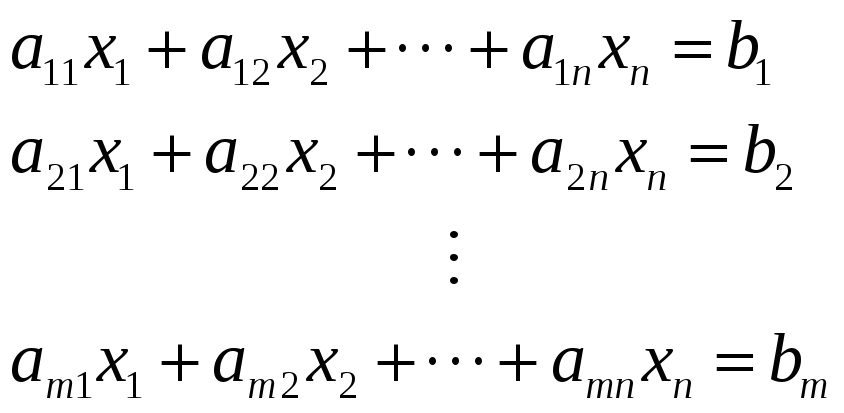the matrix representation is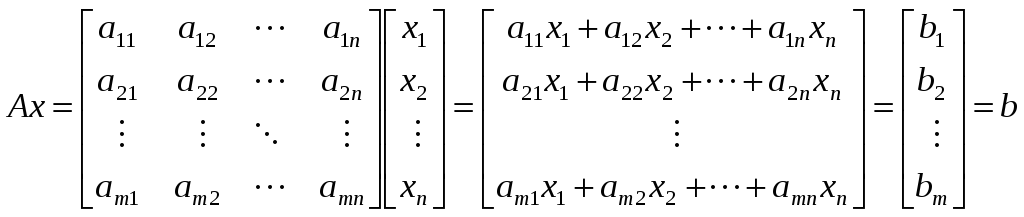,where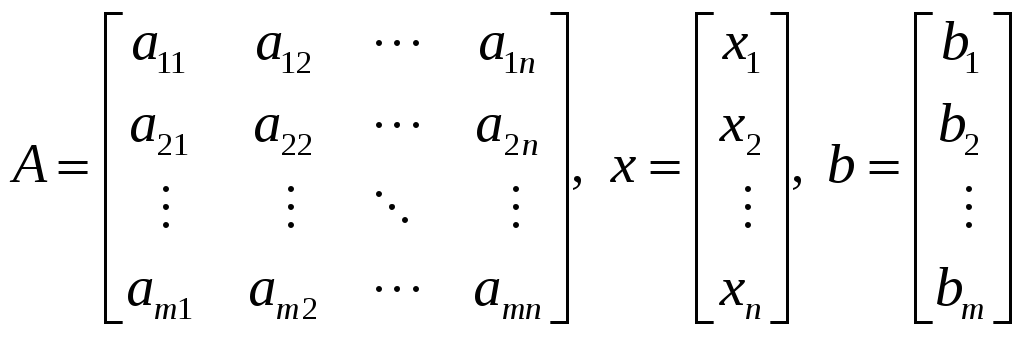Example: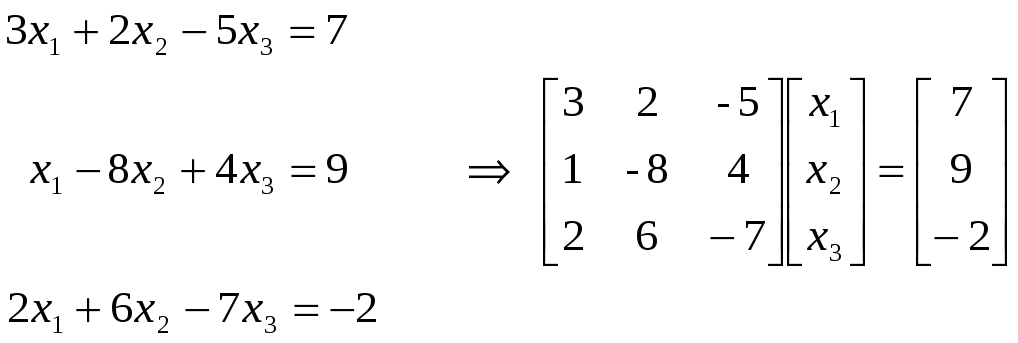.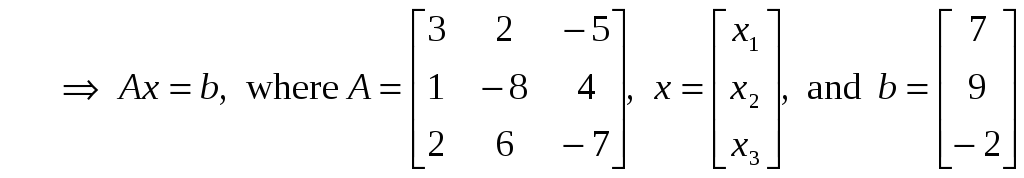. Download 1.8 Mb.Share with your friends: